# Solutions: Topics in Pre-Algebra

#### Order of Operations

Exercise Problem Solution
1. 9 + 6 x (8 - 5) 9 + 6 x (8 - 5)
= 9 + 6 x 3
= 9 + 18
= 27
2. (14 - 5) ÷ (9 - 6) (14 - 5) ÷ (9 - 6)
= 9 ÷ (9 - 6)
= 9 ÷ 3
= 3
3. 5 x 8 + 6 ÷ 6 - 12 x 2 5 x 8 + 6 ÷ 6 - 12 x 2
= 40 + 6 ÷ 6 - 12 x 2
= 40 + 1 - 12 x 2
= 40 + 1 - 24
= 41 - 24
= 17
4.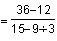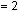5. A caterer charges a setup fee of \$50, plus \$20 per person. How much will the caterer charge if 35 people attend the party, and the customer has a coupon for \$100 off the total? 50 + 20 x 35 - 100
= 50 + 700 - 100
= 750 - 100
= 650

#### Order of Operations With Exponents

 Exercise Problem Solution 1. 32 x 43 32 x 43 = 9 x 43 = 9 x 64 = 576 2. 27 - 256 ÷ 43 27 - 256 ÷ 43 = 27 - 256 ÷ 64 = 27 - 4 = 23 3. 9 x (5 + 3)2 - 144 9 x (5 + 3)2 - 144 = 9 x 82 - 144 = 9 x 64 - 144 = 576 - 144 = 432 4. 7 + 3 x 24 ÷ 6 7 + 3 x 24 ÷ 6 = 7 + 3 x 16 ÷ 6 = 7 + 48 ÷ 6 = 7 + 8 = 15 5. A carpenter charges \$10 per square foot to lay a floor. If a square-shaped hallway is 6 feet along one side, and the customer has a coupon for \$25 off the total, then how much will the floor cost? 10 x 62 - 25 = 10 x 36 - 25 = 360 - 25 = 335 Answer: \$335

#### Order of Operations With Integers

Exercise Problem Solution
1. 18 - (-6)2 ÷ 9 18 - (-6)2 ÷ 9
= 18 - 36 ÷ 9
= 18 - 4
= 14  or  +14
2. [49 + 3(4-9)] ÷ -17 [49 + 3(4-9)] ÷ -17
= [49 + 3(-5)] ÷ -17
= [49 + -15] ÷ -17
= 34 ÷ -17
= -2
3. 53 + (-9)(+2) ÷ 6 53 + (-9)(+2) ÷ 6
= 125 + (-9)(+2) ÷ 6
= 125 + -18 ÷ 6
= 125 + -3
= 122  or  +122
4.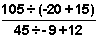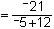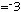5. (-4)(-3) ÷ 6 - 2[5 - -8 + (6 ÷ 2)] (-4)(-3) ÷ 6 - 2[5 - -8 + (6 ÷ 2)]
= (-4)(-3) ÷ 6 - 2[5 - -8 + 3]
= (-4)(-3) ÷ 6 - 2[13 + 3]
= (-4)(-3) ÷ 6 - 2
= (-4)(-3) ÷ 6 - 32
= 12 ÷ 6 - 32
= 2 - 32
= -30

#### Writing Algebraic Expressions

 Exercise Problem Solution 1. Fifteen less than twice a number 2x - 15 2. Three times a number increased by seventeen 3a + 17 3. The product of nine and a number, decreased by six 9m - 6 4. Thirty divided by seven times a number None of the above. 5. Jenny earns \$30 a day working part time at a supermarket. Write an algebraic expression to represent the amount of money she will earn in d days. 30d

#### Writing Algebraic Equations

 Exercise Problem Solution 1. Twice a number is twenty-eight. 2x = 28 2. The quotient of thirty-nine and a number is three.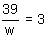3. Five less than three times a number is forty-six. 3t - 5 = 46 4. Three is twenty-one divided by the sum of a number and five. None of the above. 5. Eric had \$197 in his savings account before he was paid his weekly salary. His current savings balance is \$429. How much money does Eric earn each week? 197 + n = 429

#### Practice Exercises

Exercise Problem Solution
1. Evaluate this arithmetic expression:
7 x 8 - 6 ÷ 2 + 18 x 3
7 x 8 - 6 ÷ 2 + 18 x 3
= 56 - 6 ÷ 2 + 18 x 3
= 56 - 3 + 18 x 3
= 56 - 3 + 54
= 53 + 54 = 107
2. Evaluate this arithmetic expression: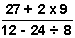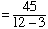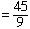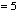3. Evaluate this arithmetic expression:
15 - 2 x 32 ÷ 6
15 - 2 x 32 ÷ 6
= 15 - 2 x 9 ÷ 6
= 15 - 18 ÷ 6
= 15 - 3
= 12
4. Evaluate this arithmetic expression:
36 ÷ (7-4)2 + 21
36 ÷ (7-4)2 + 21
= 36 ÷ 32 + 21
= 36 ÷ 9 + 21
= 4 + 21
= 25
5. Evaluate this arithmetic expression:
[54 - 3(2 + 4)] ÷ -9
[54 - 3(2 + 4)] ÷ -9
= [54 - 3(6)] ÷ -9
= [54 - 18] ÷ -9
= 36 ÷ -9
= -4
6. Evaluate this arithmetic expression: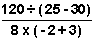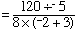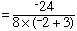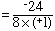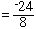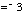7. Which algebraic expression correctly represents this phrase?
Twenty-five less than three times a number.
3x - 25
8. Which algebraic expression correctly represents this phrase?
The quotient of a number and seven, decreased by ten.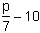9. Which sentence correctly represents this algebraic equation?
2w - 9 = 32
Nine less than twice a number is thirty-two.
10. Which sentence correctly represents this algebraic equation?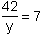The quotient of forty-two and a number is seven.

#### Challenge Exercises

Exercise Problem Solution
1. An agent charges \$150 per gig to book a rock band, plus \$75 per month for travel expenses. What was his monthly fee if he booked 6 gigs for the band last month? 150 x 6 + 75
= 900 + 75
= 975
2. A gardener charges \$8 per square foot to lay sod. If a square garden is 7 feet along each side, how much will he charge to lay sod on it? 8 x 72
= 8 x 49
= 392
3. Six people in a club will share the expenses of a party that costs \$240. How much will Katie pay for her share of the party if the club also credits her \$8? 240 ÷ 6 - 8
= 40 - 8
= 32
4. Evaluate the following expression: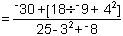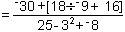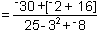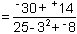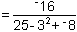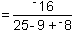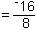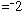5. Jesse spends \$5 a day on lunch. Which algebraic expression correctly represents the amount of money he will spend on lunch in x days? 5x
6. Which algebraic expression correctly represents this phrase?
The quotient of twelve and seven times a number, decreased by five7. Which algebraic equation correctly represents this sentence?
A number increased by eight is nineteen.
y + 8 = 19
8. Which algebraic equation correctly represents this sentence?
Twenty-five is three times a number, decreased by eight.
25 = 3w - 8
9. Which sentence correctly represents this algebraic equation?
15 = 16y - 3
None of the above.
10. Which sentence correctly represents this algebraic equation?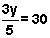The quotient of three times a number and five is thirty.